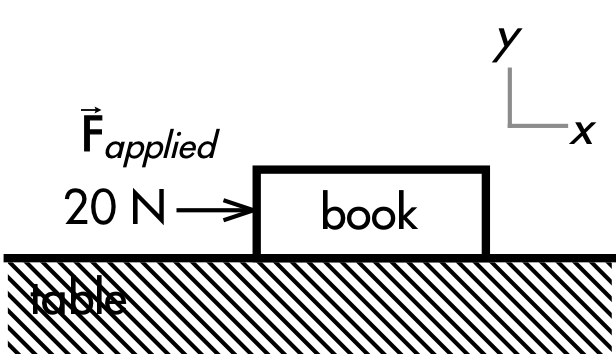## 20171003

### Physics quiz question: horizontal forces on book sliding at constant speed

Physics 205A Quiz 3, fall semester 2017
Cuesta College, San Luis Obispo, CAA force of 20 N is applied to the right on a 1.5 kg book such that it continues to slide to the right with constant speed. The table is not frictionless. Newton's __________ law tells you that these two forces are equal in magnitude and opposite in direction:
Applied force on the book.
Kinetic friction force of the table on the book.
(A) first.
(B) second.
(C) third.
(D) (These forces are not equal in magnitude and/or opposite in direction.)

Correct answer (highlight to unhide): (A)

The book has two vertical forces acting on it:
Weight force of Earth on book (downwards).
Normal force of book on suitcase (upwards).
Because the book has no vertical motion, these vertical two forces are equal in magnitude and opposite in direction, due to Newton's first law, such that these two forces produce a vertical net force of zero.

The book has two horizontal forces acting on it:
Kinetic friction force of floor on book (to the left, against the direction of motion).
External applied force on book (20 N, to the right).
Because the book is moving at constant speed (to the right), then its horizontal acceleration is zero, and by Newton's first law these two horizontal forces are equal in magnitude and opposite in direction in order to produce a horizontal net force of zero.

Sections 70854, 70855
Exam code: quiz03T4uC
(A) : 16 students
(B) : 14 students
(C) : 8 students
(D) : 16 students

Success level: 30%
Discrimination index (Aubrecht & Aubrecht, 1983): 0